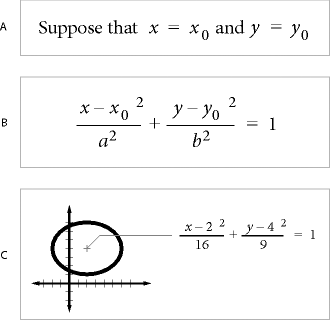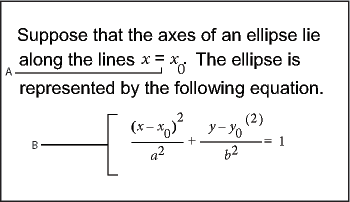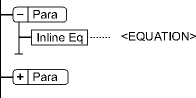## Equations

### Placing and displaying equations

You can place an equation inline with paragraph text or display it in a paragraph of its own. In either case, FrameMaker creates an anchored frame for the equation. You can position an equation with other objects in an existing graphic frame, to annotate a graphic, for example. The graphic frames that contain equations can be either anchored or unanchored.A. Inline B. Display C. In a graphic with other objects

#### Inline and display equations in structured documents

An equation element provides a frame with an equation object into which you can enter mathemat­ical expressions. Like an anchored frame for graphics, an equation frame is anchored to a specific location in text.

You can insert an equation element inline with paragraph text or in a separate display paragraph.A. Inline equation B. Display equation

An equation element appears in the document structure where it is anchored to text, regardless of the equation position on the page. In the Structure View, a square-cornered bubble with the snippet <EQUATION> indicates an equation element. The equation itself is not part of the structure.

Equation elementIf you export to SGML or XML, FrameMaker writes the equation to a separate graphics file (CGM format). Then FrameMaker adds an entity reference to it from your SGML or XML file.

You can change the file format for equations using a read/write rule. If you change the file format to MIF, FrameMaker keeps a track of equations and their respective file names. Saving the equation multiple times, still saves it to its one file, instead of creating multiple files as in FrameMaker 9.

For example:

New behavior

Old behavior

Equation 1 is saved in file say eq1.mif

Editing the FrameMaker content and subsequently saving the file, saves equation 1 to the same eq1.mif.

FrameMaker does NOT create new files on every save for the same equation.

Equation 1 is saved in file say eq1.mif

Editing the FrameMaker content and subsequently saving the file, creates a new file say eq2.mif for the same equation 1.

FrameMaker creates new files on every save for the same equation.

#### Equations in graphic frames in structured documents

You can also place an equation in an anchored frame that’s already inserted for graphics. Do this to combine the equation with graphic objects. If the anchored frame is an element, the element is part of the document structure. However, the frame contents, including the equation, do not appear in the structure.

An equation in an anchored frame with graphics sometimes produces unwanted results if you export to SGML or XML. In general, if you plan to export to SGML or XML, place only one object in a frame.

September 30, 2016